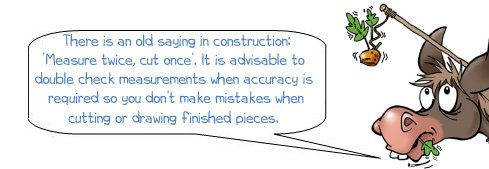# How to measure accurately with a rule

Shop for Rules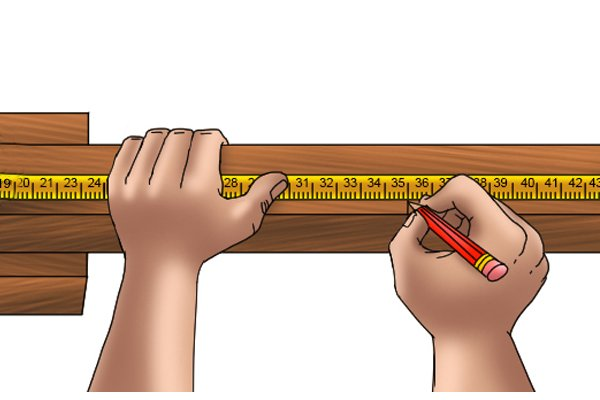A good quality rule should be accurate to its smallest gradation. If used properly, rules can be used to make fairly precise measurements.

Rules usually come with metric or imperial measurements, or both. This means they are usually marked in metres, centimetres and millimetres, or feet, inches and divisions of inches, depending on their size and design. Most rules have metric gradations down one edge and imperial gradations down the other.

## How to read metric measurements with a rule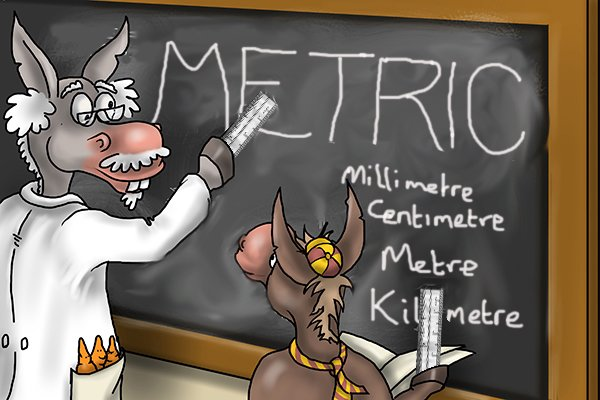Metric measurements are generally easier to work with and record than imperial as it is divided decimally, which means it’s divided by tens. Metric is the official measuring system of all the countries in the world, apart from America, Liberia and Burma.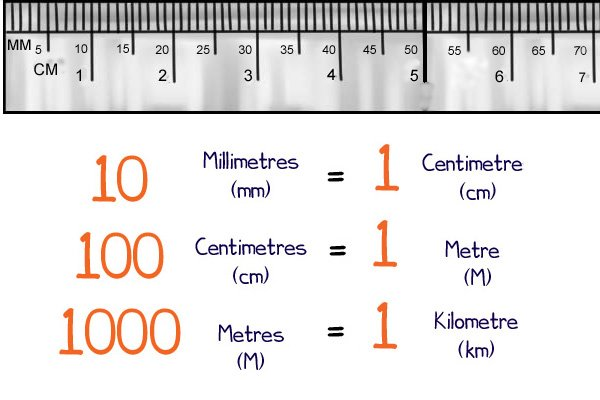Metric rules usually have the larger gradations in centimetres and each centimetre is divided into 10 millimetres. Some technical rules may also have half millimetres marked out. Metric measurements are represented with decimal numbers, rather than fractions. So a measurement read as four millimetre marks past 5 cm would be read as 5.4 cm. To get the millimetres this number is multiplied by 10, so 5.4 cm is 54 mm. Longer rules will also have metres marked out.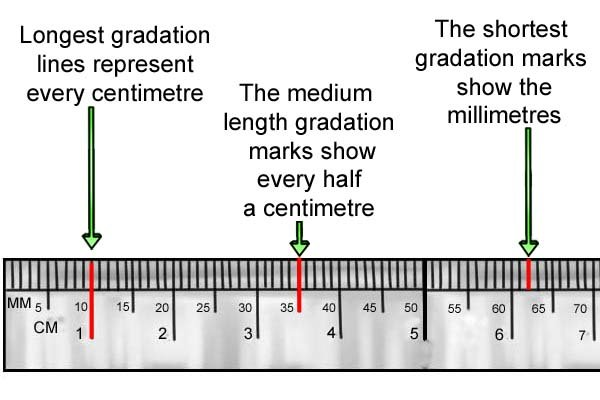To make reading a rule easier, the gradation marks are usually different lengths. For metric rules to longest marks represent each metre (if the rule is long enough). The second longest marks, or the longest ones on smaller rules, represent centimetres (or ten mm). The next longest lines will be every half a centimetre (every 5 mm). The smallest lines on most rules will represent millimetres. If half millimetres are shown they will be the smallest lines.

## How to read imperial measurements with a rule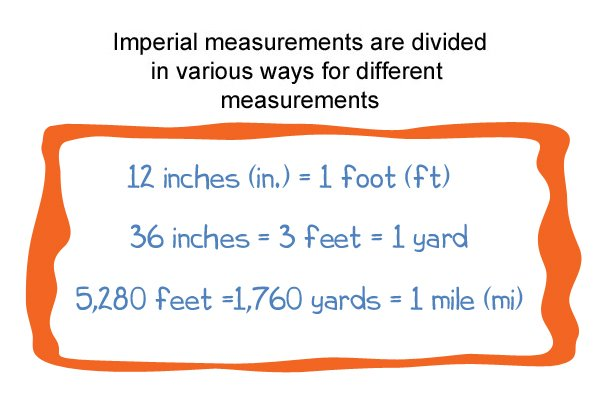Imperial measurements are not as easy to read as metric measurements because they are not divided into multiples of ten. There are three feet in a yard, twelve inches in a foot (single of feet) and inches are divided into 1/2 inch, 1/4 inch, 1/8 inch, 1/16 inch or 1/32 inch, with some being divided into 1/64 inch. In most places, imperial measurements have been superseded by metric measurements altogether.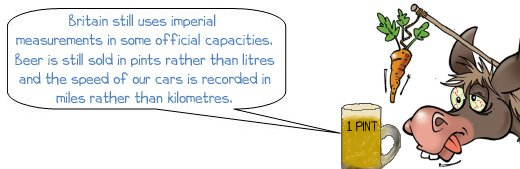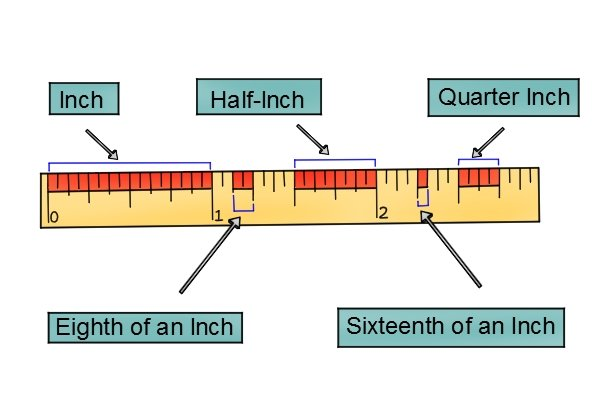If you are using the imperial measurements it’s probably worth noting that the inches on different rules may be divided differently. There are also some specialist imperial rules which are divided into 1/10 inch. The longest gradation marks will be inches; the smaller the divisions get the shorter the gradation lines will be.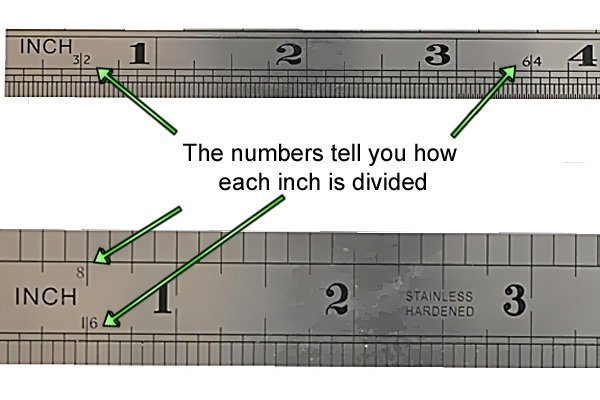Most rules will state what the smallest divisions of the inch are. If they don’t, a simple way to determine how the gradations are divided is to count the marks between the whole measurements. Inches are read and represented as fractions, unlike metric measurements which are decimals. So if the rule was divided into 1/8 inch and you read the measurement at one mark past the two inch mark you would write the measurement as 2 1/8 inch, not 2.125 inch.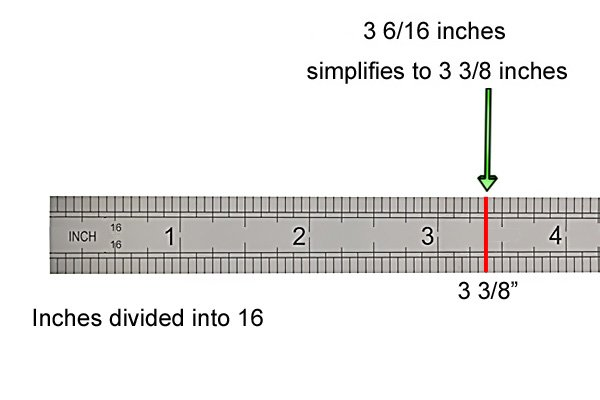To measure with imperial units, you would take the number of the whole inch plus the number of fraction gradations. You then need to simplify the fraction. On a 1/16 inch divided rule if the measurement was six gradation marks after the three inch mark it would be 3 6/16 inches, which would then be simplified to 3 3/8 inches.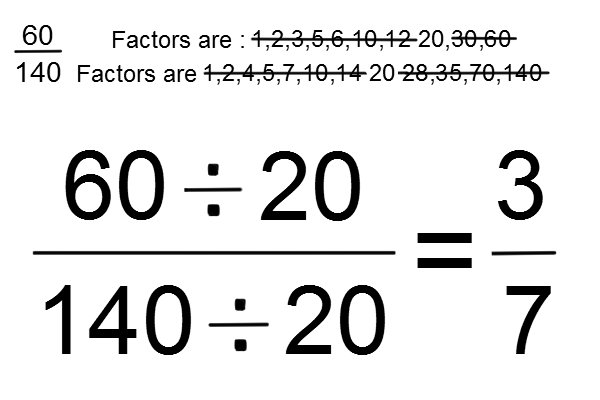To simplify a fraction you need to look at the two numbers which make up that fraction, then find the largest number both the fraction numbers can be divided by, and still produce a whole number in each case. Divide the fraction numbers, separately, by that number and a simplified fraction should be produced.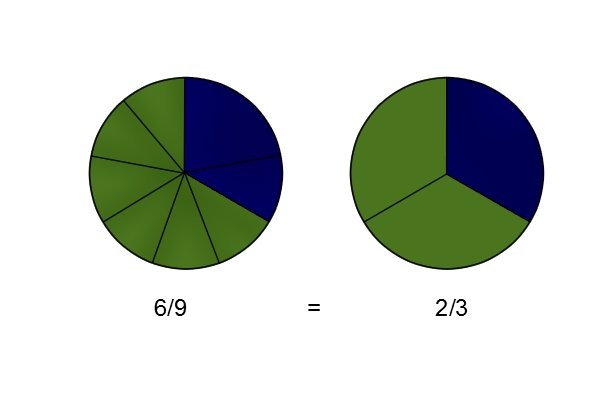So if the fraction is 6/9, the highest number they can both be divided by is three. Six divided by three is two and nine divided by three is three, so the simplified fraction becomes 2/3. If the fraction was 7/8 the largest number they could both be divided by is one, therefore, the fraction is already simplified as much as it can be. The same applies for larger fractions: 32/64 can be simplified down to 1/2, because the thirty-two is the largest number they can both be divided by.

## How to reduce inaccuracies when reading a rule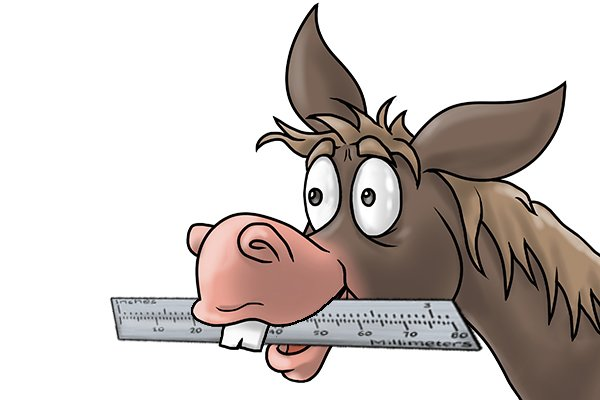The first thing that will determine how accurate a rule is will be the quality of the rule. Cheaper rules are likely to have a higher degree of inaccuracy than more expensive rules. There are also other factors which can increase the inaccuracy of a rule.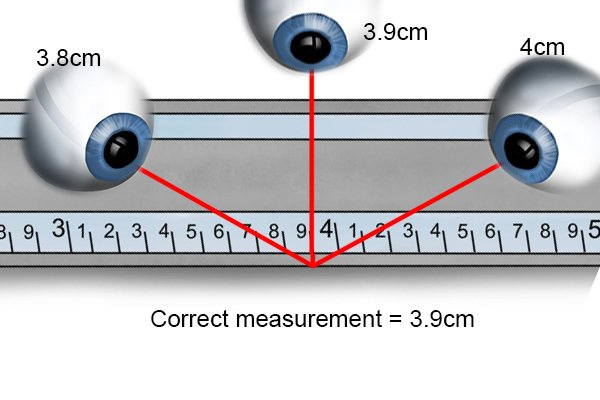### Parralax

Measurements can easily be misread on a rule if they are not looked at correctly. The most common cause of user error when reading rules is called parallax. This is the distortion of a viewed object depending on the angle it is being view from. The thicker a rule is the more chance there is that  parallax will affect the reading when it’s laid flat on a surface. This is because of the distance between the surface you’re measuring and the gradation marks you’re reading.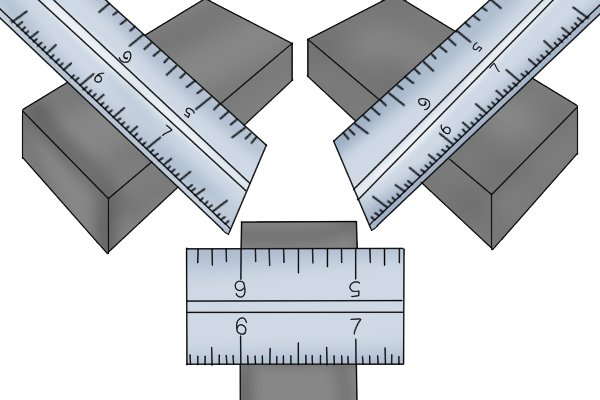The most accurate measurements are made when the gradation lines are as close to the surface being measured as possible and they are viewed straight on. The rule needs to have a straight, undamaged edge to be accurate. If a rule is read from an angle, the reading can be slightly wrong. If the rule is bent, the edge is not completely straight, or it is damaged, the measurement reading will not be precise and it’s advisable to replace the rule.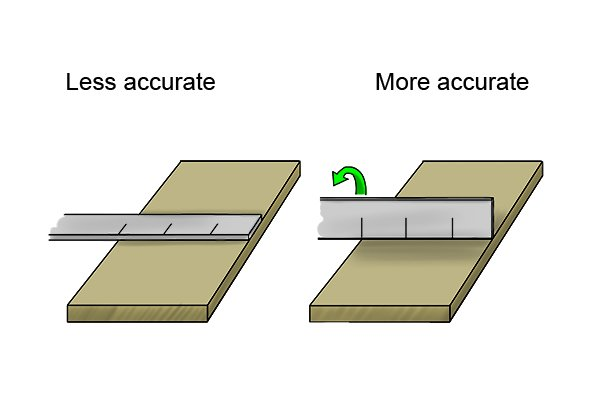To get the gradation marks of a rule as close to the surface you’re measuring as possible, the rule can be stood on its side, with the reading edge on the surface and the gradation marks adjacent to it. If the rule and surface are straight there should be no gap between them. You still need to make sure you read the rule straight on from this position to be as accurate as possible.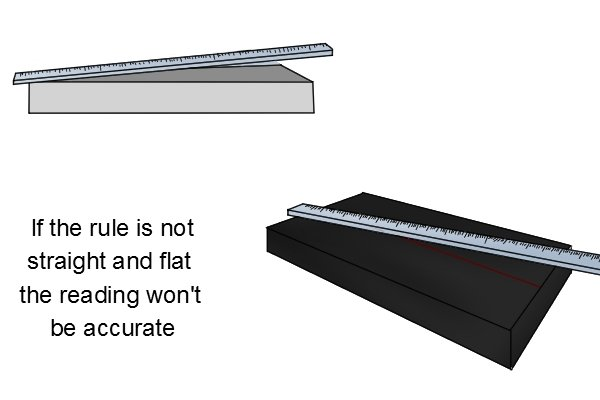### Positioning the rule

Where you actually place the rule while measuring can also affect the accuracy.

You need to make sure the rule is straight across the dimension you’re measuring and lying flat. If the rule is placed at an angle, the measurements will not be correct.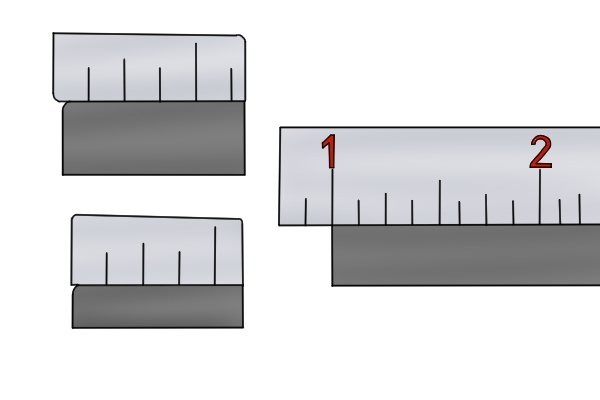If possible, take measurements between gradation lines, rather than from the end of the rule. However, be sure to make allowances for where you are measuring from, and don’t just read the number on the line. Measurements taken from the end of a rule can be less accurate as this relies heavily on how accurately the end of the rule has been formed. You can measure from the end of a better quality rule if there is a hard stop the rule can be butted against.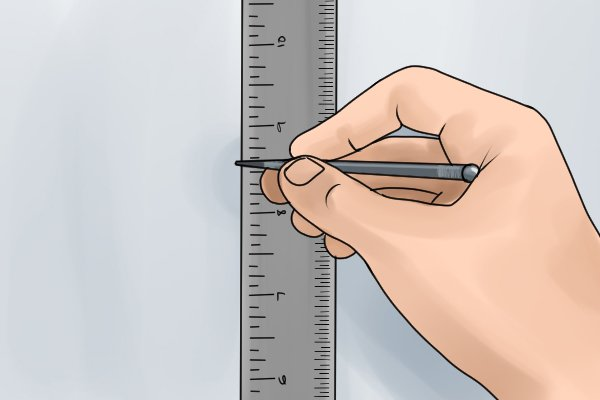Remember that the gradation lines themselves have a width measurement. Measurements taken from one side of a gradation line will be slightly different if measured from the other side of the line. To get the most accurate measurement it’s advisable to use a thin pencil or pen tip and mark the measurement in the centre of the gradation line.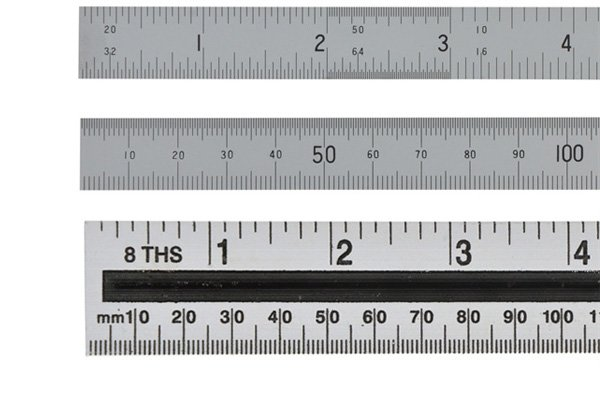Which gradation marks you take the measurement from will depend on how accurate you need to be. For detailed work, that needs to be precise, you will need to use the smallest gradation marks on the rule. If the measurements do not need to be exact then the measurements can be rounded up or down, using the larger gradation separations, such as centimetres or half centimetres.

## Measuring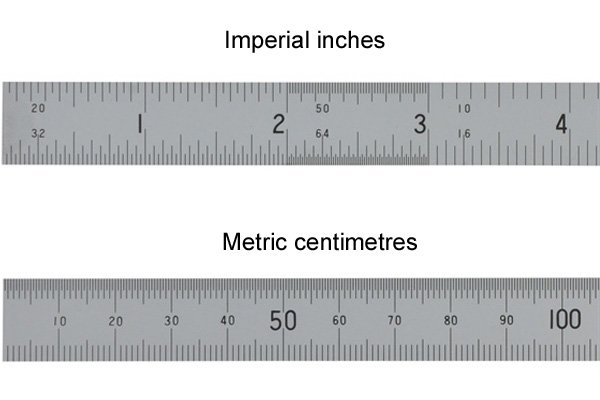### Step 1 –  Choose a measurement system

If there are gradation marks in imperial and metric measurements you will need to determine which you want to use. It is advisable to just use one system of measurements for an entire project, which should ensure accuracy for dimensions being measured and save having to use conversions.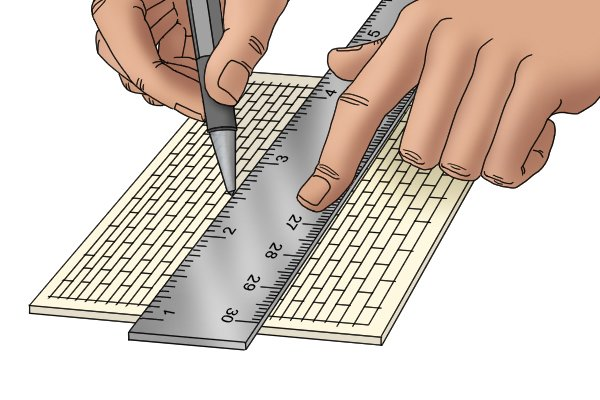### Step 2 – Position rule

Following the guidelines above to reduce inaccuracy, position the rule on the distance you want to measure. Make sure you hold it still and straight when taking the reading.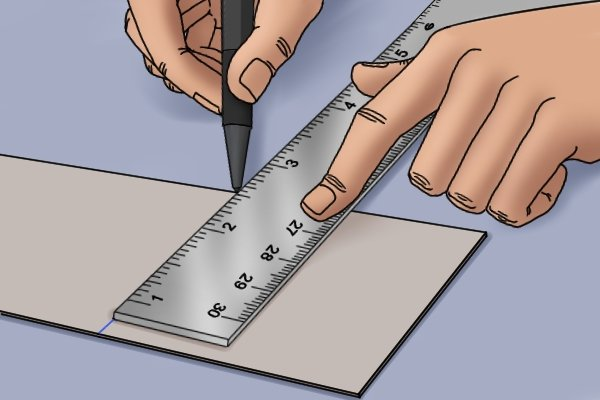### Step 3 – Read measurement

Read the measurement by finding the first whole unit. This will generally be whole centimetres or whole inches. You then read the marks after that whole measurement to find the millimetres or fractions of inches.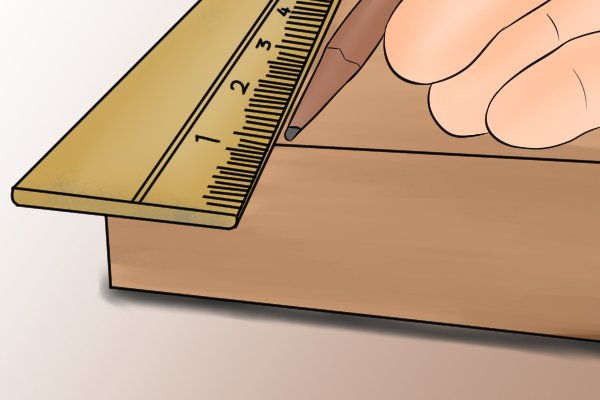If you are starting the measurement at the zero mark on the rule, you simply read the mark the end of the measurement falls at. If you have started at a gradation up from the zero mark then subtract the number you started with from the final measurement. For example, if you took a measurement that started at the 1 cm mark and finished at the 11 cm mark the final measurement will be 10 cm (11-1).